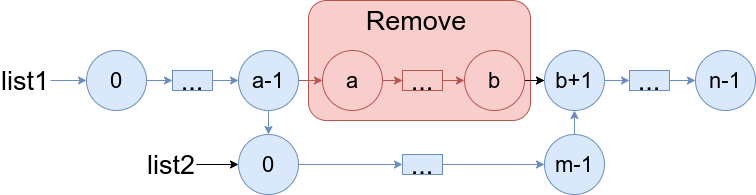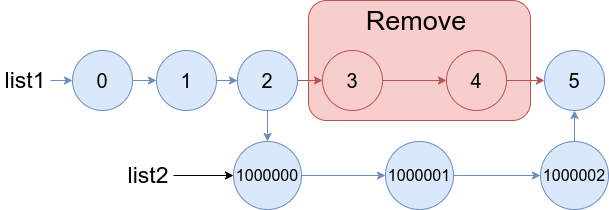1669. Merge in Between Linked Lists

# 1669. Merge In Between Linked Lists#

## 题目 #

You are given two linked lists: `list1` and `list2` of sizes `n` and `m` respectively.

Remove `list1`'s nodes from the `ath` node to the `bth` node, and put `list2` in their place.

The blue edges and nodes in the following figure incidate the result:Build the result list and return its head.

Example 1:``````Input: list1 = [0,1,2,3,4,5], a = 3, b = 4, list2 = [1000000,1000001,1000002]
Output: [0,1,2,1000000,1000001,1000002,5]
Explanation: We remove the nodes 3 and 4 and put the entire list2 in their place. The blue edges and nodes in the above figure indicate the result.

``````

Example 2:``````Input: list1 = [0,1,2,3,4,5,6], a = 2, b = 5, list2 = [1000000,1000001,1000002,1000003,1000004]
Output: [0,1,1000000,1000001,1000002,1000003,1000004,6]
Explanation: The blue edges and nodes in the above figure indicate the result.

``````

Constraints:

• `3 <= list1.length <= 104`
• `1 <= a <= b < list1.length - 1`
• `1 <= list2.length <= 104`

## 解题思路 #

• 简单题，考查链表的基本操作。此题注意 a == b 的情况。

## 代码 #

``````func mergeInBetween(list1 *ListNode, a int, b int, list2 *ListNode) *ListNode {
n := list1
var startRef, endRef *ListNode
for i := 0; i <= b; i++ {
if i == a-1 {
startRef = n
}
if i == b {
endRef = n
}
n = n.Next
}
startRef.Next = list2
n = list2
for n.Next != nil {
n = n.Next
}
n.Next = endRef.Next
return list1
}
``````Apr 8, 2023Edit this page